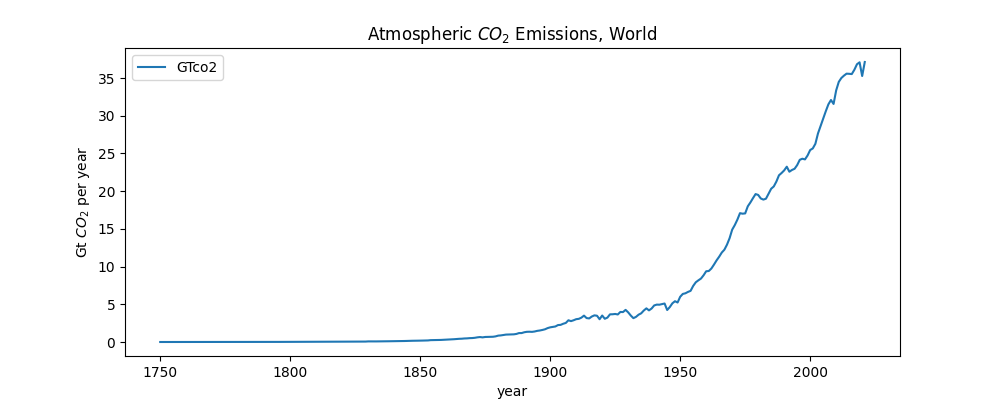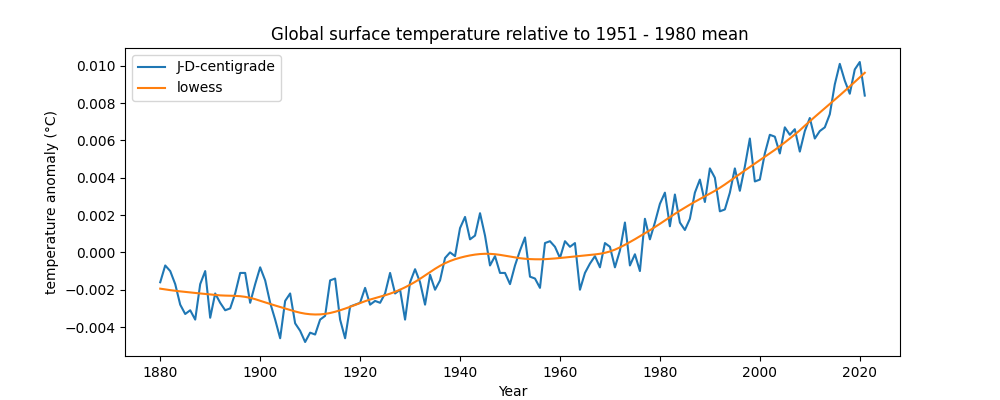Computer Science

Material in Unit 6 is optional. You are not expected to know this for a test and there are no labs or projects using this material.

# Using pandas to plot climate change data

This guide shows how to use pandas to plot climate change data. If you are new to pandas, see introduction to pandas.

This guide is NOT designed to give you a thorough introduction to plotting with pandas. It uses some more advanced techniques. Rather, I just want to show you some real-world applications of pandas using a topic I am passionate about. That’s a great way to learn how to plot in Python — find something you’re interested in and then dive in.

This notebook is inspired by the R hockeystick library, which makes it really easy to plot some common climate change graphs.

You can view a Google Colab notebook of this guide page.

## BYU Forum from Dr. Katharine Hayhoe

To gain some context on the importance of climate change, watch this BYU forum from Dr. Katharine Hayhoe. Dr. Hayhoe is a renowned climate scientist and a Christian. She gave this talk in fall semester 2022 and received a standing ovation. Dr. Hayhoe explained how being a Christian means loving all of God’s creations. This led her to become a climate scientist and she believes addressing climate change is “a true expression of God’s love.”

## CO2 Emissions

We are first going to plot worldwide $CO_2$ emissions. This dataset includes years and the $CO_2$ emitted each year, for each country. We are going to look at just the worldwide data.

import pandas as pd

# CO2 data can be found at
# https://github.com/owid/co2-data

# An explanation of columns is at
# https://github.com/owid/co2-data/blob/master/owid-co2-codebook.csv

def plot_global_co2_per_year(csv_filename):

# convert the CSV file into a Pandas dataframe

# get the subset of the data where the country column is equal to 'World'
world = data[data["country"].eq("World")]

# create a new column where co2 is measured in gigatons instead of megatons
world["co2"].div(1000)

# create a line plot with x = year and y = GTco2
# set the figure size, title, xlabel, ylabel
plot = world.plot.line(
x="year",
y="co2",
figsize=(10, 4),
title="Atmospheric $CO_2$ Emissions, World ",
xlabel="year",
ylabel="Gt $CO_2$ per year",
)

# get a figure for the plot
fig = plot.get_figure()

# save the figure to a file
fig.savefig("global-co2-per-year.png")

if __name__ == "__main__":
plot_global_co2_per_year("owid-co2-data.csv")

This creates the following plot:Some of the things you see in the code above include:

• data = pd.read_csv('https://raw.githubusercontent.com/owid/co2-data/master/owid-co2-data.csv') — We can load datasets over the Internet.

• world = data[data["country"].eq("World")] — This gets a subset of the data where the country column is equal to World. The dataset contains data for every country, but we just want to look at the worldwide data.

• world["co2"].div(1000) — This divides the entire co2 column by 1000, so we can convert megatons to gigatons. It modifies the column instead of returning a new dataframe.

• $CO_2$ — This uses LaTeX math notation, which is valid in CoLab notebooks and in strings when plotting.

## Global temperature anomalies

Next we are going to plot land-surface air and sea water temperature anomalies. This data shows how much the temperature of the earth (including both air and sea) has warmed relative to a mean of the data from 1951 to 1980.

We are going to plot both the raw data — the anomaly per year — and a curve that fits the data with a LOWESS smoothing, which is a form of regression analysis. This can show a smoothed trend over time.

import pandas as pd

# pip install statsmodels
from statsmodels.nonparametric.smoothers_lowess import lowess

# Global Land-Surface Air and Sea Water Temperature Anomalies
# (Land-Ocean Temperature Index, L-OTI)
# This has the global surface temperature relative to 1951-1980 mean
# https://data.giss.nasa.gov/gistemp/

# The specific file used here is
# https://data.giss.nasa.gov/gistemp/tabledata_v4/GLB.Ts+dSST.csv

# The text file contains some additional explanation
# https://data.giss.nasa.gov/gistemp/tabledata_v4/GLB.Ts+dSST.txt

def plot_global_loti_per_year(csv_filename):

# convert the CSV file into a Pandas dataframe
# we need to skip the first row!

# the column labeled J-D is the anomaly for that entire year
# the data description says to divide by 100 to convert to centigrade

# skip years where annual average not computed
data = data[data["J-D"] != "***"]

# create a new column where the anomaly is measured in degrees centigrade
# must convert column to a float first, since Pandas sees it as a string

# create a line plot with x = year and y = J-D-centigrade
# set the figure size, title, xlabel, ylabel
ax = data.plot.line(
x="Year",
figsize=(10, 4),
title="Global surface temperature relative to 1951 - 1980 mean ",
xlabel="year",
ylabel="temperature anomaly (°C)",
)

# get x and y values for LOWESS
x = data['Year'].values

# run LOWESS
y_hat = lowess(y, x, frac=1/5)

# add LOWESS to data frame
data['lowess'] = y_hat[:,1]

data.plot(ax=ax, x='Year', y='lowess')

# get a figure for the plot
fig = ax.get_figure()

# save the figure to a file
fig.savefig("global-loti-per-year.png")

if __name__ == "__main__":
plot_global_loti_per_year("GLB.TS+dSST.csv")

This creates the following plot:Some of the things you see in the code above include:

• data = data[data["J-D"] != "***"] — We are using the J-D column because this has data averaged for the whole year, January through December. We are going to ignore any data that is incomplete, which is marked in this dataset with ***.

• data["J-D-centigrade"] = data["J-D"].astype(float).div(100) — This creates a new column where the anomaly is measured in degrees centigrade. We first use astype() to convert the column to a float, since Pandas sees it as a string. This is because values start with - for negative numbers.

• We want to compute the LOWESS smoothing using a numpy array. numpy is a library that provides fast scientific computation for Python.

• x = data['Year'] and y = data['J-D-centigrade'] — Gets separate columns for x and y values for the LOWESS smoothing.

• y_hat = lowess(y, x, frac=1/5) — Calculates the LOWESS smoothing, using the x and y values and a parameter frac equal to 1/5 — the lower the value, the greater the smoothing. The lowess function comes from a statsmodels library. It returns a two-dimensional array of (x, y) values.

• data['lowess'] = y_hat[:,1] — This adds a column with the y values of the LOWESS calculation to the dataframe. The notation y_hat[:,1] says to get all the rows, and the first column, from the y_hat array.

• data.plot(ax=ax, x='Year', y='lowess') — This adds an additional line plot onto our previous plot. The ax = ax sets the axis to the the same as the previous plot.

## Global Warming Stripes

We now create a plot of ‘warming stripes’, which were created by Professor Ed Hawkins, a climate scientist. You can create your own stripes at #ShowYourStripes.

import pandas as pd

# Be sure to run:
# pip install matplotlib
import matplotlib.pyplot as plt
from matplotlib.patches import Rectangle
from matplotlib.collections import PatchCollection
from matplotlib.colors import ListedColormap

# code from
# https://matplotlib.org/matplotblog/posts/warming-stripes/

# This data is published by the Met Office in the UK. It shows
# combined sea and land surface temperatures as +/- from the average for
#the years 1961 to 1990.

# An explanation of the columns is here:

# first and last years in the dataset
FIRST = 1850
LAST = 2021

# Reference period for the center of the color scale
FIRST_REFERENCE = 1971
LAST_REFERENCE = 2000
LIM = 0.7 # degrees

def global_warming_stripes(fwf_filename):
# read a fixed-width file (fwf) into a Pandas data frame
# a fixed-width file has file formatted into columns using spaces

# index_col --  which column to use for row labels
# usecols --  which columns to use from the file
# names --  how to name the columns
# header -- indicates there is no first row with column names
data = pd.read_fwf(fwf_filename, index_col=0, usecols=(0, 1),

# create a new table that has all the columns where the year is between
# FIRST and LAST, dropping any that don't have data
data = data.loc[FIRST:LAST, 'anomaly'].dropna()

# take the mean of the anomaly data for the years between FIRST_REFERENCE
# and LAST_REFERENCE
reference = data.loc[FIRST_REFERENCE:LAST_REFERENCE].mean()

# create a set of colors for the bars
# the colors in this colormap come from http://colorbrewer2.org
# the 8 more saturated colors from the 9 blues / 9 reds

cmap = ListedColormap([
'#08306b', '#08519c', '#2171b5', '#4292c6',
'#6baed6', '#9ecae1', '#c6dbef', '#deebf7',
'#fee0d2', '#fcbba1', '#fc9272', '#fb6a4a',
'#ef3b2c', '#cb181d', '#a50f15', '#67000d',
])

# create a figure , giving its size
fig = plt.figure(figsize=(10, 1))

# add axes -- the dimensions are [left, bottom, width, height]
ax = fig.add_axes([0, 0, 1, 1])
# turn off showing lines, ticks, labels, etc for the axes
ax.set_axis_off()

# create a collection of rectangles, one for each year
rectangles = []
for year in range(FIRST, LAST + 1):
# create a Rectangle with (x, y) starting point, width, height
rectangles.append(Rectangle((year, 0), 1, 1))

collection = PatchCollection(rectangles)

# set data, colormap and color limits for the collection
collection.set_array(data)
collection.set_cmap(cmap)
collection.set_clim(reference - LIM, reference + LIM)

# set the limits on the axes
ax.set_ylim(0, 1)
ax.set_xlim(FIRST, LAST + 1)

# save the figure
fig.savefig('global-warming-stripes.png')

if __name__ == '__main__':
global_warming_stripes('HadCRUT.4.6.0.0.annual_ns_avg.txt')

This creates the following plot:Some of the things you see in the code above include:

• import matplotlib.pyplot as plt — We import various items from the matplotlib library. This is a low level, and thus very powerful, library for plotting in Python.

• pd.read_fwf() — we use read_fwf to read the dataset because the file is NOT stored as a CSV, it is stored as a fixed-with file, meaning the columns are all a fixed width, using spaces.

• data = data.loc[FIRST:LAST, 'anomaly'].dropna() — This gets certain rows from the dataframe, dropping any that are missing data.

• reference = data.loc[FIRST_REFERENCE:LAST_REFERENCE].mean() — We are going to be plotting bars of various colors. So we use this to get the mean of the anomaly for the period 1971 — 2000. We will use this as the middle temperature and plot colder temperatures in shades of blue, with warmer temperatures in red.

• cmap = ListedColormap(...) — This creates a colormap, meaning a list of colors, using their hex values.

• fig = plt.figure(figsize=(10, 1)) — Creates a blank figure of a given size.

• ax = fig.add_axes([0, 0, 1, 1]) — This adds an axis object to the figure, which is a container for the X, Y axes, the plot, the legend, and so forth. A plot can contain multiple “axes”, meaning it can have multiple subfigures. See here for a good visualization.

• Rectangle((year, 0), 1, 1) — Each bar on the chart has a size, starting from the (year, 0) point in (x, y) space, and extending 1 unit high and 1 unit wide.

• PatchCollection(rectangles) — This is a collection of rectangles, so we can operate on them all at once.

• collection.set_array(data) — This maps each rectangle in the collection to one of the anomaly values from our dataset.

• collection.set_cmap(cmap) — This provides our colormap (a list of colors) to the collection of rectangles.

• collection.set_clim(reference - LIM, reference + LIM) — This maps each rectangle to a color. From above, each rectangle is associated with an anomaly value. This command indicates that low range for the anomaly (reference - LIM) is set to the first color in the map, and the high range for the anomaly (reference + LIM) is set to the last color in the map. The rest are colors in between.

• ax.add_collection(collection) — This adds all of the rectangles to the plot.